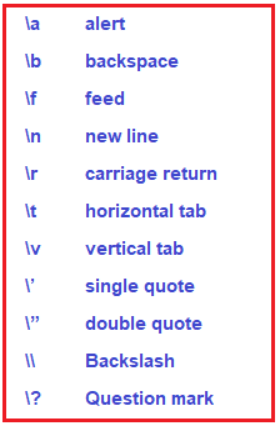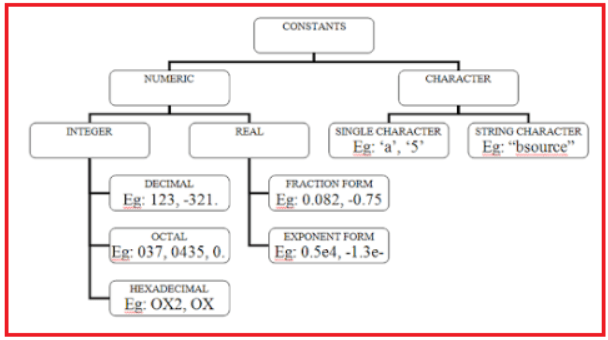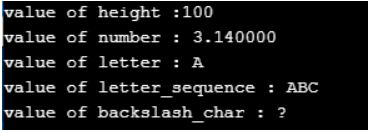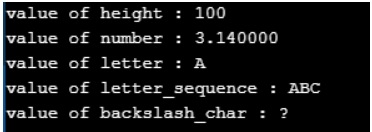# Constants in C

## Constants in C Language with Examples

In this article, I am going to discuss Constants in C Language with Examples. Please read our previous article, where we discussed the different parts of a C program. As part of this article, you will learn two things one is Character set and the other one is C Constants.

##### Character set in C

Character set refers to the characters that are recognized and accepted in C Language. C recognizes

• All alphabets from A-Z & a-z.
• All digits from 0 to 9.
• Special characters such as #,*, /, &, <, >, (,), {,}, ; etc.
• Space characters such as blank spaces and tab spaces etc.
• Backslash character or escape sequences##### Constants in C Language

Constants are the terms that cannot be changed during the execution of a program. These fixed values are also called literals. A “constant” is a number, character, or character string that can be used as a value in a program.

Example: 1, 2.5, “C Programming”, etc.

##### C Constants can be classified as:
• Integer constant
• Real constant
• Character constant
• String constant##### Integer Constant in C Language:

An integer constant is the numeric constant (constant associated with numbers) without any fractional part or exponential part. Integer constants are always positive until we specify a negative (-) sign. It must have at least one digit. It must not have any decimal point. In between the integer constant we do not have any separators including comma (,). There are three types of integer constants in the C language:

Decimal constant (base 10)
Decimal Digits: 0 1 2 3 4 5 6 7 8 9
Example: 0. -9, 22 etc.

Octal constant (base 8)
Octal Digits: 0 1 2 3 4 5 6 7
Example: 021, 077, 033 etc.

Hexadecimal Digits: 0 1 2 3 4 5 6 7 8 9 A B C D E F
Example: 0x7f, 0x2a, 0x521 etc.

Range of integer constants is: -32,768 to 32,767.

We can use small caps a, b, and c instead of uppercase letters while writing a hexadecimal constant. Ever Octal constant stars with 0 and hexadecimal constant starts with 0x in C Programming.

##### Real Constant in C Language:

Any signed or unsigned number with the fractional part is called a real constant. It is also called a Floating-Point constant. Real constants are the numeric constants that have either fractional form or exponential form. It can be positive or negative but by default it is positive. The real constant must have at least one digit. It must have a decimal point. No comma and blank space are allowed within a real constant.

The range of Real Constants is -3.4*1038 to 3.4*1038.
Decimal Form: Example: -2.0, 0.0000234,
Exponential Form: Example:-0.22E-5 (Here, E-5 represents 10-5. Thus, -0.22E-5 = -0.0000022)

##### Character Constant:

A character constant is a single digit, single alphabet, or a single special symbol. It must be enclosed within single quotes. Both the inverted commas should point to the left. We use the escape character along with the character. The escape character says that it is not a normal character and does something. The maximum length can be 1 character. The value of a character constant is the numerical value of a character in the matching character set for example in the ASCII character set, character zero has a numerical value of 48 which is not at all related to the numerical value 0.

Example: ‘A’, ‘5’.
The range of Character Constants is -128 to 127.

##### String Constant:

String Constant is a sequence of characters enclosed between double-quotes. The character may be letters, numbers, special characters, and blank spaces. For example (“HelloWorld”), here you can see HelloWorld is a sequence of characters that is enclosed between double quotes (“ ”) so, this is a String constant. A string constant is an array of characters with a null character at the end of the string.

Example: “Hello”, “1987”, “?….!”, “x”, ” “, “” (null string constant).

##### How to use Constants in a C Program?

We can define constants in a C program in the following ways. By const keyword and by #define preprocessor directive. If you try to change constant values after defining in C program, it will throw you an error.

##### Example using const keyword in C Language:
```#include <stdio.h>
void main ()
{
const int height = 100;	/*int constant */
const float number = 3.14;	/*Real constant */
const char letter = 'A';	/*char constant */
const char letter_sequence = "ABC";	/*string constant */
const char backslash_char = '\?';	/*special char constant */
printf ("value of height :%d \n", height);
printf ("value of number : %f \n", number);
printf ("value of letter : %c \n", letter);
printf ("value of letter_sequence : %s \n", letter_sequence);
printf ("value of backslash_char : %c \n", backslash_char);
}```
###### Output:##### Example using #define preprocessor directive in C:
```#include <stdio.h>
#define height 100
#define number 3.14
#define letter 'A'
#define letter_sequence "ABC"
#define backslash_char '\?'
void main ()
{
printf ("value of height : %d \n", height);
printf ("value of number : %f \n", number);
printf ("value of letter : %c \n", letter);
printf ("value of letter_sequence : %s \n", letter_sequence);
printf ("value of backslash_char : %c \n", backslash_char);
}```
###### Output:In the next article, I am going to discuss Operators in C Language with examples. Here, in this article, I try to explain Constants in C Language with Examples and I hope you enjoy this Constants in C Language with Examples article.